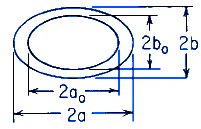Related Resources: calculators

### Torsional Deformation Stress Hollow Elliptical Section Equation and Calculator

Beam Deflection and Stress Equation and Calculators

Torsional Deformation and Stress Hollow Elliptical Section Equations and Calculator. Hollow elliptical section, outer and inner boundaries similar ellipsesALL calculators require a Premium Membership

Preview

Torsional Deformation and Stress Eccentric Hollow Elliptical Section CalculatorWhere:

q = ao / a = bo / b

Wall thickness is not constant

Angle of Twist under applied Torque Moment

θ = ( T L ) / ( K G )

Shear Stress

τmax = 2 T / [ π a b2 ( 1 - q4 ) ] at end of monor axis on outer surface

Where:

θ = angle of twist (radians)
α = degrees
T = Twisting or torque moment force-length, (in-lbs, N-mm)
L = Length (in, mm)
a, b = Diameter (in, mm)
τ = Unit shear stress force / area (lbs/in2, N/mm2)
G = Modulus of rigidity force / area (lbs/in2, N/mm2)
K = Polar Moment of Inertia (in4, mm4) for section

Reference:
Roarks Formulas for Stress and Strain, 7th Edition, Table 10.1 Formulas for torsional deformation and stress.